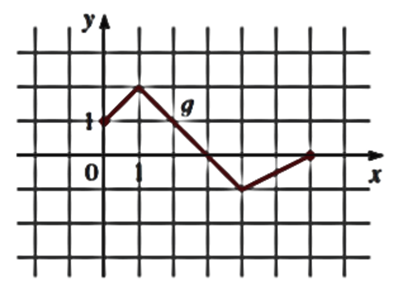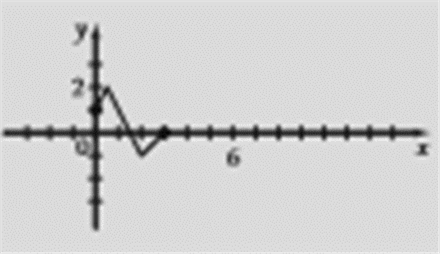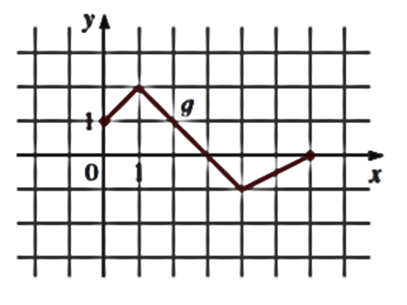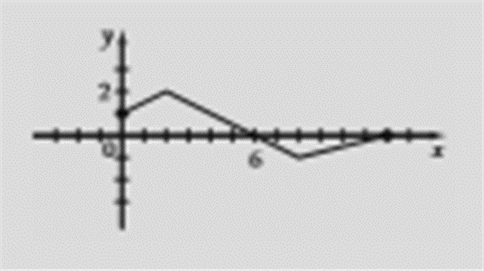# To sketch the graph of the function.### Precalculus: Mathematics for Calcu...

6th Edition
Stewart + 5 others
Publisher: Cengage Learning
ISBN: 9780840068071### Precalculus: Mathematics for Calcu...

6th Edition
Stewart + 5 others
Publisher: Cengage Learning
ISBN: 9780840068071

#### Solutions

Chapter 2.5, Problem 65E

a.

To determine

## To sketch the graph of the function.

Expert Solution

### Explanation of Solution

Given information:

The function is y=g(x)

Graphthe graph obtained as:Interpretation: from the above graph it is observed that when y=g(x) is given, the graph of the function y=g(2x) is obtained by horizontal stretching the graph of y=g(x) by the factor of 2 .

b.

To determine

Expert Solution

### Explanation of Solution

Given information:

The function is y=g(x)Graph :

The graph obtained as:Interpretation: from the above graph it is observed that when y=g(x) is given, the graph of the function y=g(12x) is obtained by horizontal shrinking the graph of y=g(x) by the factor of 12 .

### Have a homework question?

Subscribe to bartleby learn! Ask subject matter experts 30 homework questions each month. Plus, you’ll have access to millions of step-by-step textbook answers!